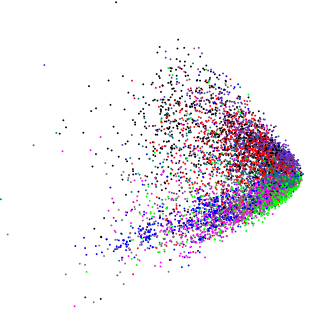# What does a wedge-like shape of the PCA plot indicate?

In their paper on autoencoders for text classification Hinton and Salakhutdinov demonstrated the plot produced by 2-dimensional LSA (which is closely related to PCA):.

Applying PCA to absolutely different slightly high dimensional data I obtained a similarly looking plot:(except in this case I really wanted to know if there is any internal structure).

If we feed random data into PCA we obtain a disk-shaped blob, so this wedge-shaped shape is not random. Does it mean anything by itself?

• I assume all the variables are positive (or non-negative) & continuous? If so, the edges of the wedge are just the points beyond which the data would become 0/negative. Further, you can get the same pattern you show with positive right-skewed variables; the observations are clumped up at the low end. If you had positive uniform random variables you'd see a (rotated) square. Hence patterns like the one you show are just constraints on the data. Other patterns can show up, like a horseshoe, but these aren't due to constraints on the ranges of the variables. – Gavin Simpson Apr 1 '16 at 18:49
• @GavinSimpson This is considerably more than a comment. Why not expand it into an answer? – Mike Hunter Apr 1 '16 at 19:24
• I asked my kids (3 and 4 years old) what do these pictures remind them and they said it's a fish. So perhaps "fish-like shape"? – amoeba Apr 1 '16 at 19:28
• @GavinSimpson, thanks! In both cases variables are indeed non-negative, bot also in both cases they are integer valued. Does this change anything? – macleginn Apr 1 '16 at 19:47

## 1 Answer

Assuming the variables are positive or non-negative the edges of the edge are are just points beyond which the data would become 0 or negative respectively. As such real-life data tend to be right skewed, we see greater density of points at the low end of their distribution and hence greater density at the "point" of the wedge.

More generally, PCA is simply a rotation of the data and constraints on those data will generally be visible in the principal components in the same manner as shown in the question.

Here is an example using several log-normally distributed variables:

library("vegan")
set.seed(1)
df <- data.frame(matrix(rlnorm(5*10000), ncol = 5))
plot(rda(df), display = "sites")Depending on the rotation implied by the first two PCs, you might see the wedge or you might see a somewhat different version, show here in 3d using (ordirgl() in place of plot())Here, in 3d we see multiple spikes protruding from the centre mass.

For Gaussian random variables ($X_i \sim \mathcal(N)(\mu = 0, \sigma = 1)$) where each has the same mean and variance we see a sphere of points

set.seed(1)
df2 <- data.frame(matrix(rnorm(5*10000), ncol = 5))
plot(rda(df2), display = "sites")And for uniform positive random variables we see a cube

set.seed(1)
df3 <- data.frame(matrix(runif(3*10000), ncol = 3))
plot(rda(df3), display = "sites")Note that here, for illustration I show the uniform using just 3 random variables hence the points describe a cube in 3d. With higher dimensions/more variables we can't represent the 5d hypercube perfectly in 3d and hence the distinct "cube" shape gets distorted somewhat. Similar issues effect the other examples shown, but it's still easy to see the constraints in those examples.

For your data, a log transformation of the variables prior to PCA would pull in the tails and stretch out the clumped data, just as you might use such a transformation in a linear regression.

Other shapes can crop up in PCA plots; one such shape is an artefact of the metric representation preserved in the PCA and is known as the horseshoe. For data with a long or dominant gradient (samples arranged along a single dimension with variables increasing from 0 to a maximum and then decreasing again to 0 along portions of the data are well known to generate such artefacts. Consider

ll <- data.frame(Species1 = c(1,2,4,7,8,7,4,2,1,rep(0,10)),
Species2 = c(rep(0, 5),1,2,4,7,8,7,4,2,1, rep(0, 5)),
Species3 = c(rep(0, 10),1,2,4,7,8,7,4,2,1))
rownames(ll) <- paste0("site", seq_len(NROW(ll)))
matplot(ll, type = "o", col = 1:3, pch = 21:23, bg = 1:3,
ylab = "Abundance", xlab = "Sites")which produces an extreme horseshoe, where points at the ends of the axes bend back into the middle.